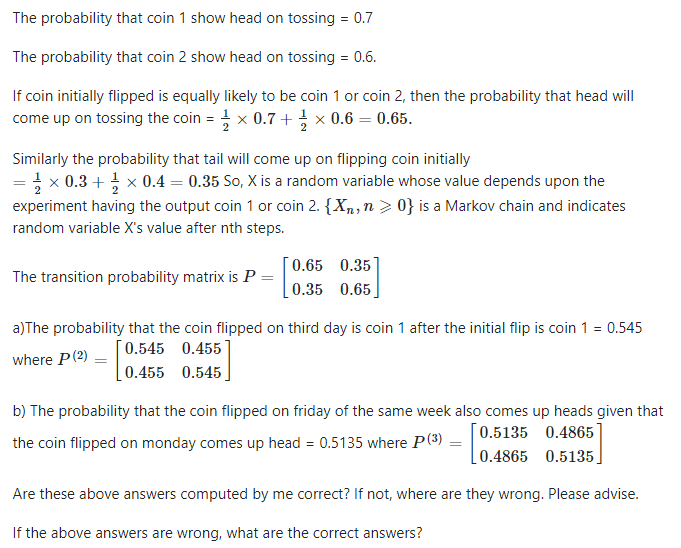# Coin flipping problem (Markov chain)

## Recommended Posts

Suppose that coin 1 has probability 0.7 of coming up heads, and coin 2 has probability 0.6 of coming up heads.

If the coin flipped today comes up heads, then we select coin 1 to flip tomorrow, and if it comes up tails, then we select coin 2 to flip tomorrow.

If the coin initially flipped is equally likely to be coin 1 or coin 2, then

a)what is the probability that the coin flipped on the third day after the initial flip is coin 1?

b)Suppose that the coin flipped on Monday comes up heads. What is the probability that the coin flipped on Friday of the same week also comes up heads?

My attempt to answer this question:##### Share on other sites

You assume to much; for instance, why would a binary random answer be greater than 50%?

##### Share on other sites

On 7/30/2022 at 10:05 AM, dimreepr said:

You assume to much; for instance, why would a binary random answer be greater than 50%?

Weighted coins? Magnets? Without symmetry why would you assume it to be 50%?

Edited by J.C.MacSwell

## Create an account

Register a new account